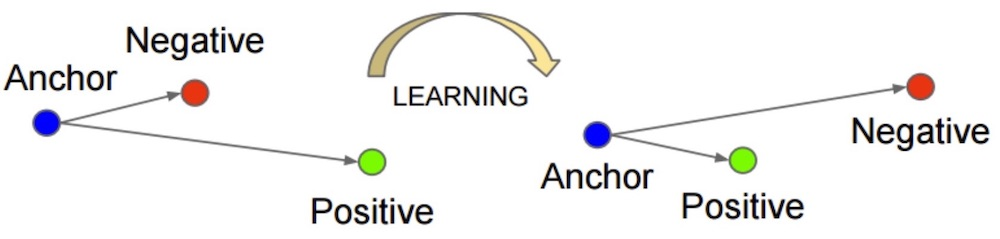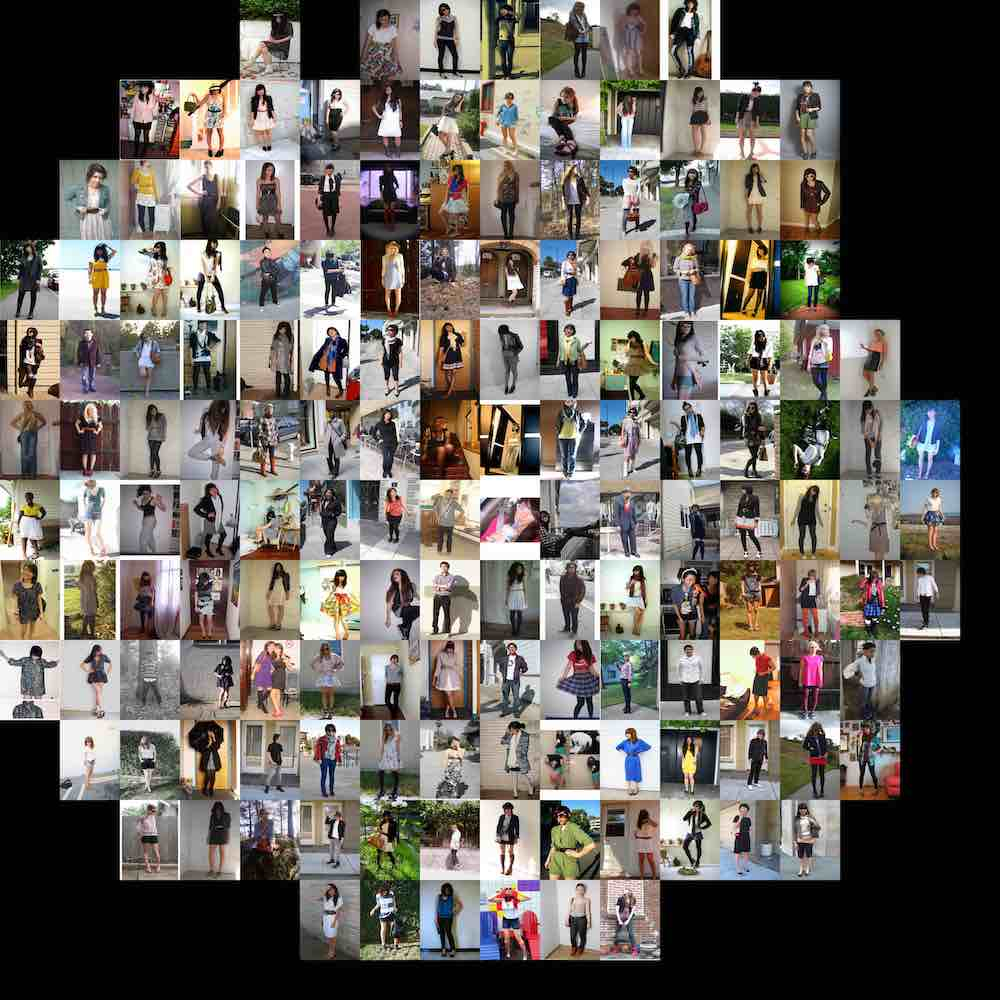Year: 2016

# Abstract

We present a system that directly learns a compact feature representation for fashion photographs taken in real world environments. The learned representation is shown to encode an understanding of fashion style despite not directly being trained to classify between fashion styles. The representation is learned through training on weakly labeled data from fashion photographs posted to online social websites. The system learns a style similarity metric by comparing whether pairs of images share attributes – colors and garments. In particular, training is performed using triplets that consist of an anchor image, a dissimilar image, and a similar image. The performance of the learned embedding is thoroughly evaluated and is shown to be generic in that it can be used on tasks it was not directly trained for, such as fashion style classification.

## Triplet Image Selection

Triplets of images are sampled by selecting an anchor image I and randomly sampling a similar image I+ and a dissimilar image I- according to similarity thresholds defined on the intersection over union of the image tags.

## Architecture and Loss

A convolutional neural network extracts a feature embedding for each image in the triplet. The Euclidean distances $\left\lVert f_- - f \right\rVert$ and $\left\lVert f_+ - f \right\rVert$ are computed between feature vector pairs. These distance are use to compute an embedding loss, either Triplet or Ranking. The objective of the embedding loss is to increase the distance in the feature embedding space between the anchor image I and the dissimilar image I- and to decrease the distance between the anchor image I and the similar image I+.The network learns a similarity metric defined on the feature embedding space, which encodes the similarity of the images with respect to fashion style.

### Ranking Loss

The ranking loss maximizes the distance $d_-$ between the anchor image I and the dissimilar image I- and minimizes the distance $d_+$ between the anchor image I and the similar image I+.
$l_E(I_-,I,I_+) = \frac{1}{2}\left((d_+)^2+ (1-d_-)^2 \right) = (d_+)^2$
where $d_+$ and $d_-$ are the softmax normalized feature distance between similar and dissimilar pairs.

$d_- = \frac{\exp\left(\left\lVert f_- - f\right\rVert_2\right)}{\exp\left(\left\lVert f_- - f\right\rVert_2\right) + \exp\left(\left\lVert f_+ - f\right\rVert_2\right)}$
$d_+ = \frac{\exp\left(\left\lVert f_+ - f\right\rVert_2\right)}{\exp\left(\left\lVert f_- - f\right\rVert_2\right) + \exp\left(\left\lVert f_+ - f\right\rVert_2\right)}$

### Triplet Loss

The triplet loss is a version of the hinge loss which encourages the distance between the anchor image I and the similar image I+ to be smaller than the distance between the anchor image I and the dissimilar image I- by at least some parameter \alpha.

### Overall Loss

A separate classification network predicts a binary presence or absence value for each tag in the dissimilar image I- given the feature vector $f_-$. The overall loss is the weighted sum of the embedding and classification losses.

## Embedding VisualizationA t-SNE visualization of images sampled from the Fashion 144k dataset, arranged using the embedding learned by the fashion network.

• TensorFlow
• Python